Win up to 100% scholarship on Aakash BYJU'S JEE/NEET courses with ABNAT Win up to 100% scholarship on Aakash BYJU'S JEE/NEET courses with ABNAT

# JEE Main 2021 March 18 – Shift 1 Maths Question Paper with Solutions

JEE Main 2021 March 18th Shift 1 Maths Question Paper can be accessed on this page. The question paper comes with step-by-step solutions that are designed by subject experts at BYJU’S. These solutions will help them to understand the correct answer and at the same time analyze their performance in the JEE Main exam. Students can easily download the JEE Main 2021 March 18th Shift 1 Maths Question Paper PDF also. In essence, practicing solving these questions will help students to study productively and improve their speed and accuracy for the upcoming exams.
JEE Main 2021 March 18th Shift 1 Maths Question Paper

Section – A

Question 1: If the functions are defined as f (x) = √x and g(x) = √(1 – x), then what is the common domain of the following functions: f + g, f – g, f / g, g / f, g – f where (f ± g) (x) = f (x) ± g (x), (f / g) (x) =

a. 0 < x ≤ 1
b. 0 ≤ x < 1
c. 0 ≤ x ≤ 1
d. 0 < x < 1

f + g = √x + √(1 – x)

x ≥ 0 and 1 – x ≥ 0 ⇒ x ∈ [0, 1]

f – g = √x – √(1 – x)

x ≥ 0 and 1 – x ≥ 0 ⇒ x ∈ [0, 1]

f / g = √x / (√1 – x)

x ≥ 0 and 1 – x > 0 ⇒ x ∈ [0, 1]

g / f = √(1 – x) / √x

1 – x ≥ 0 and x > 0 ⇒ x ∈ [0, 1]

g – f = √(1 – x) – √x

1 – x ≥ 0 and x ≥ 0 ⇒ x ∈ [0, 1]

x ∈ (0, 1)

Question 2: Let α, β, γ be the roots of the equations, x3 + ax2 + bx + c = 0, (a, b, c ∈ R and a, b and a, b ≠ 0). The system of the equations (in u, v, w) given by αu + βv + γw = 0; βu + γv + αw = 0; γu + αv + βw = 0 has non-trivial solutions, then the value of a2 / b is

a. 5
b. 1
c. 0
d. 3

x3 + ax2 + bx + c = 0

For non-trivial solutions,

$$\begin{array}{l}\begin{vmatrix} \alpha &\beta &\gamma \\ \beta&\gamma &\alpha \\ \gamma &\alpha &\beta \end{vmatrix}=0\end{array}$$

α3 + β3 + γ3 – 3αβγ = 0

(α + β + γ) (α + β + α2 – 3 ∑αβ) = 0

(–a) [a2 – 3b] = 0

a2 = 3b [because a ≠ 0]

a2 / b = 3

Question 3: If the equation a

$$\begin{array}{l}a|Z|^2+ \bar{\alpha}Z+\alpha \bar{Z}+d=0\end{array}$$
represents a circle where a, d are real constants, then which of the following condition is correct?

a. |α|2 – ad ≠ 0
b. |α|2 – ad > 0 and a ∈ R – {0}
c. α = 0, a, d ∈ R+
d.|α|2 – ad ≥ 0 and a ∈ R

$$\begin{array}{l}z\overline{z}+(\alpha /a)\overline{z}+[\overline {\alpha}/a]z+(d/a)=0\\\end{array}$$

Centre = – α / a

r = √(|α / a|2 – (d / a))

(|α / a|2 ≥ (d / a))

Question 4: 1 / (32 – 1) + 1 / (52 – 1) + 1 / (72 – 1) + ……. + 1 / (2012 – 1) is equal to:

a. 101 / 404
b. 101 / 408
c. 99 / 400
d. 25 / 101

S = ∑r=1100 [1 / [(2r + 1)2 – 1]] = ∑r=1100 1 / [(2r + 2) (2r)]

S = (1 / 4) ∑r=1100 [(1 / r) – (1 / (r + 1))]

S = (1 / 4) [(1 – (1 / 2)) + ((1 / 2) – (1 / 3)) + ((1 / 3) – (1 / 4) …… ((1 / 100) – (1 / 101))

S = (1 / 4) (100 / 101)

= 25 / 101

Question 5: The number of integral values of m so that the abscissa of point of intersection of lines 3x + 4y = 9 and y = mx + 1 is also an integer, is:

a. 3
b. 2
c. 1
d. 0

3x + 4(mx + 1) = 9

x(3 + 4m) = 5

x = 5 / (3 + 4m)

(3 + 4m) = ± 1, ± 5

4m = – 3 ± 1, –3 ± 5

4m = – 4, –2, –8, 2

m = –1, – 1 / 2, – 2, 1 / 2

Two integral value of m

Question 6: The solutions of the equation

$$\begin{array}{l}\begin{vmatrix} 1+sin^{2}x &sin^{2}x &sin^{2}x \\ cos^{2}x &1+cos^{2}x &cos^{2}x \\ 4sin2x&4 sin2x &1+4sin2x \end{vmatrix}=0\end{array}$$
, (0 < x < π), are:

a. π / 6, 5π / 6
b. 7π / 12, 11π / 12
c. 5π / 12, 7π / 12
d. π / 12, π / 6

$$\begin{array}{l}\\R_{1}\rightarrow R_{1}+R_{2}\\ \begin{vmatrix} 2 &2 &1 \\ cos^{2}x&1+cos^{2}x &cos^{2}x \\ 4sin2x&4sin2x &1+4sin2x \end{vmatrix}=0\\ C_{1}\rightarrow C_{1}-C_{2}\\ \begin{vmatrix} 0 &2 &1 \\ -1&1+cos^{2}x &cos^{2}x \\ 0&4sin2x &1+4sin2x \end{vmatrix}=0\\\end{array}$$

∴ 2 + 8 sin 2x – 4 sin 2x = 0

⇒ sin 2x = – 1 / 2

⇒ x = 7π / 12, 11π / 12

Question 7: If

$$\begin{array}{l}\left\{\begin{matrix} 1/|x|, &x\geq 1 \\ ax^{2}+b,&|x|<1 \end{matrix}\right.\end{array}$$
is differentiable at every point of the domain, then the values of a and b are respectively:

a. 5 / 2, – 3 / 2
b. – 1 / 2, 3 / 2
c. 1 / 2, 1 / 2
d. 1 / 2, – 3 / 2

f (x) is continuous at x = 1 ⇒ 1 = a + b

f (x) is differentiable at x = 1 ⇒ –1 = 2a

a = – 1 / 2, b = 3 / 2

Question 8: A vector a has components 3p and 1 with respect to a rectangular Cartesian system. This system is rotated through a certain angle about the origin in the counterclockwise sense. If with respect to the new system, a has components p + 1 and √10, then a value of p is equal to:

a. 1
b. –1
c. 4 /5
d. – 5 / 4

|a|old = |a|new

(3p)2 + 1 = (P + 1)2 + 10

9p2 – p2 – 2p – 10 = 0

8p2 – 2p – 10 = 0

4p2 – p – 5 = 0

4p2 – 5p + 4p – 5 = 0

(4p – 5) (p + 1) = 0

p = 5 / 4, –1

Question 9: The sum of all the 4-digit distinct numbers that can be formed with the digits 1, 2, 2 and 3 is:

a. 26664
b. 122664
c. 122234
d. 22264

1 2 2 3

1 2 3 2

1 3 2 2

3 1 2 2

3 2 1 2

3 2 2 1

2 1 3 2

2 3 1 2

2 2 1 3

2 2 3 1

2 3 2 1

2 1 2 3

Sum = 2 6 6 6 4

Question 10: Choose the correct statement about two circles whose equations are given below:

x2 + y2 – 10x – 10y + 41 = 0

x2 + y2 – 22x – 10y + 137 = 0

a. circles have no meeting point
b. circles have two meeting points
c. circles have only one meeting point
d. circles have the same centre

Let S1 : x2 + y2 – 10x – 10y + 41 = 0

⇒ (x – 5)2 + (y – 5)2 = 9

Centre (C1) = (5, 5)

S2 : x2 + y2 – 22x – 10y + 137 = 0

⇒ (x – 11)2 + (y – 5)2 = 9

Centre (C2) = (11, 5)

Distance (C1 C2) = √(5 – 11)2 + (5 – 5)2

Distance (C1 C2) = 6

r1 + r2 = 3 + 3 = 6

∴ The circles touch externally.

Hence, the circle has only one meeting point.

Question 11: If α, β are natural numbers such that 100α – 199β = (100) (100) + (99) (101) + (98) (102) + …. + (1) (199), then the slope of the line passing through (α, β) and origin is:

a. 510
b. 550
c. 540
d. 530

RHS = ∑r=099 (100 – r) (100 + r)

(100)3 – [(99 * 100 * 199) / 6] = 1003 – (1650) (199)

LHS = (100)α – (199)β

So, α = 3, β = 1650

Slope = tan θ = b / a

tan θ = 550

Question 12: The value of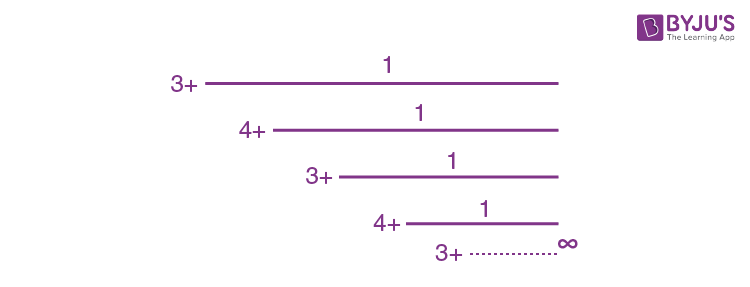is equal to:

a. 3 + 2√3
b. 4 + √3
c. 2 + √3
d. 1.5 + √3

Let y = 3 + 1 / [4 + (1 / y)]

y = 3 + y / (4y + 1)

4y2 + y = 12y + 3 + y

4y2 – 12y – 3 = 0

y = [12 ± √144 + 48] / 8

y = [12 ± 8 √3] / 8

y = [3 ± 2√3] / 2

y = [1.5 ± √3]

y = [1.5 + √3]

Question 13: The integral ∫ [(2x – 1) cos √(2x – 1)2 + 5) / (√4x2 – 4x + 6)] dx is equal to (where c is a constant of integration)

a. (1 / 2) sin √(2x + 1)2 + 5 + c
b. (1 / 2) sin √(2x – 1)2 + 5 + c
c. (1 / 2) cos √(2x + 1)2 + 5 + c
d. (1 / 2) cos √(2x – 1)2 + 5 + c

∫ [(2x – 1) cos √(2x – 1)2 + 5) / (√4x2 – 4x + 6)] dx

Put (2x – 1)2 + 5 = t2

2(2x – 1) dx = 2tdt

∫ (cos t/ t) (t / 2) dx = (1 / 2) sin t + c

= (1 / 2) sin √[(2x – 1)2 + 5] + c

Question 14: The differential equations satisfied by the system of parabolas y2 = 4a (x + a) is:

a. y (dy / dx) + 2x (dy / dx) – y = 0
b. y (dy / dx)2 + 2x (dy / dx) – y = 0
c. y (dy / dx)2 – 2x (dy / dx) – y = 0
d. y (dy / dx)2 – 2x (dy / dx) + y = 0

y2 = 4a(x + a) …… (1)

2yy’ = 4a

∴ yy’ = 2a

By (1), y2 = 2yy’ [x + (yy’ / 2)]

y2 = 2yy’x + (yy’)2

⇒ y(y’)2 + 2xy’ – y = 0 (as y ≠ 0)

Question 15: The real-valued function f (x) = cosec-1 x /√(x – [x]) where [x] denotes the greatest integer less than or equal to x, is defined for all x belonging to:

a. all non- integers except the interval [–1, 1]
b. all integers except 0, –1, 1
c. all reals except integers
d. all reals except the interval [–1, 1]

f (x) = cosec-1 x / (√x – [x])

x ∈ (- ∞, – 1] ⋃ [1, ∞) – all integers

& {x} ≠ 0 and x ≠ integer

x ∈ (- ∞, – 1) ⋃ (1, ∞) – all integers

Question 16: If limx→0 [sin-1 x – tan-1 x] / 3x3 is equal to L, then the value of (6L + 1) is:

a. 1 / 2
b. 2
c. 1 / 6
d. 6

limx→0 [sin-1 x – tan-1 x] / 3x3

L = limx→0 [x + (x3 / 6) + ….. ] – [x – (x3 / 3) + ……] / 3x3

L = (1 / 3) [(1 / 6) + (1 / 3)] = 1 / 6

(6L + 1) = 6 (1 / 6) + 1 = 2

Question 17: For all four circles M, N, O and P, the following four equations are given:

Circle M : x2 + y2 = 1

Circle N : x2 + y2 – 2x = 0

Circle O : x2 + y2 – 2x – 2y + 1 = 0

Circle P : x2 + y2 –2y = 0

If the centre of circle M is joined with the centre of the circle N, further centre of circle N is joined with the centre of the circle O, centre of circle O is joined with the centre of circle P and lastly, the centre of circle P is joined with the centre of circle M, then these lines form the sides of a:

a. Rectangle
b. Square
c. Parallelogram
d. Rhombus

CM = (0, 0)

CN = (1, 0)

CO = (1, 1)

CP = (0, 1)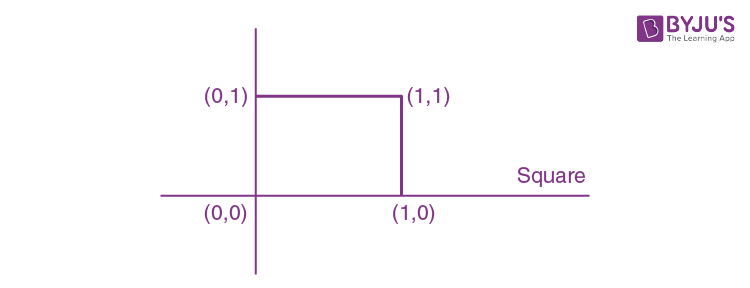Question 18: Let (1 + x + 2x2)20 = a0 + a1x + a2x2 + …… + a40x40. Then, a1 + a3 +a5 + ….+ a37 is equal to

a. 220(220 + 21)
b. 219(220 + 21)
c. 220(220 – 21)
d. 219(220 – 21)

Put x = 1, – 1 and subtract

420 – 220 = (a0 + a1 + ….. + a40) – (a0 – a1 + …….)

⇒ 420 – 220 = 2 (a1 + a3 + ….. + a39)

⇒ a1 + a3 + ….. + a37 = 239 – 219 – a39

a39 = coeff of x39 in (1 + x + 2x2)20 = 20C1 219

⇒ a1 + a3 + …… + a37 = 239 – 219 –20(219)

= 239 – 21 (219) = 219(220 – 21)

Question 19: Let

$$\begin{array}{l}A+2B=\begin{bmatrix} 1&2 &0 \\ 6&-3 &3 \\ -5&3 &1 \end{bmatrix}\end{array}$$
and
$$\begin{array}{l}2A-B=\begin{bmatrix} 2&-1 &5 \\ 2&-1 &6 \\ 0&1 &2 \end{bmatrix}\end{array}$$
. If Tr(A) denotes the sum of all diagonal elements of the matrix A, then Tr(A) – Tr(B) has value equal to:

a. 0
b. 1
c. 3
d. 2

tr (A + 2B) ≡ tr (A) + 2 tr (B) = –1 …..(1)

and tr (2A – B) ≡ 2tr (A) – tr (B) = 3 …..(2)

On solving (1) and (2) we get

tr (A) = 1, tr(B) = –1

tr (A) – tr(B) = 1 + 1 = 2

Question 20: The equations of one of the straight lines which pass through the point (1, 3) and make an angle tan-1 √2 with the straight line, y + 1 = 3√2x is:

a. 5 √2x + 4y – 15 + 4√2 = 0
b. 4 √2x – 5y – 5 + 4√2 = 0
c. 4 √2x + 5y – 4√2 = 0
d. 4 √2x + 5y – (15 + 4√2) = 0

tan (tan-1 √2) = |(m – 3√2) / (1 + 3m√2)|

√2 = |(m – 3√2) / (1 + 3m√2)|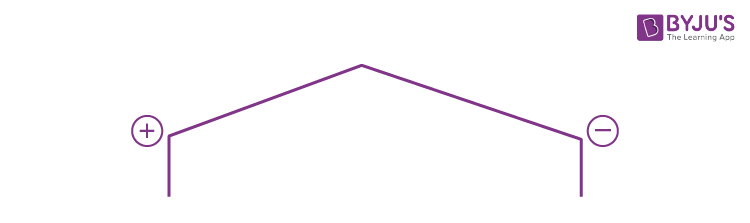6m + √2 = m – 3√2

5m = – 4 √2

m = – 4√2 / 5

– 6m – √2 = m – 3√2

2√2 = 7m

According to the options, choose, m = -2√2 / 7

y = -4√2x/5 + (3+4√2)/5

4√2 x + 5y – (15+4√2)=0

SECTION – B

Question 21: The number of times digit 3 will be written when listing the integers from 1 to 1000 is ______.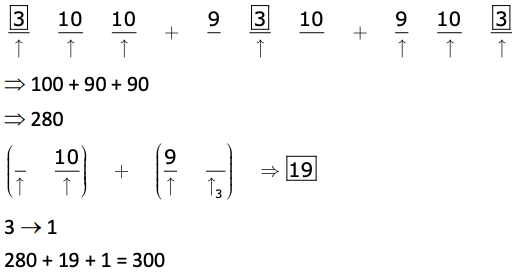Question 22: The equation of the planes parallel to the plane x – 2y + 2z – 3 = 0 which are at unit distance from the point (1, 2, 3) is ax + by + cz + d = 0. If (b – d) = K (c – a), then the positive value of K is ______.

x – 2y + 2z + λ = 0

Now given

d = |1 – 4 + 6 + λ| / √9 = 1

|λ + 3| = 3

λ + 3 = ± 3

λ = 0, – 6

So planes are: x – 2y + 2z – 6 = 0

x – 2y + 2z = 0

b – d = –2 + 6 = 4

c – a = 2 – 1 = 1

[b – d] / [c – a] = k

k = 4

Question 23: Let f (x) and g (x) be two functions satisfying f (x2) + g (4 – x) = 4x3 and g (4 –x) + g(x) = 0, then the value of ∫-44 f (x2) dx is ______.

I = 2 ∫04 f (x2) dx ……….(1)

I = 2 ∫04 f ((4 – x)2) dx ……….(2)

2I = 2 ∫04 [f (x2) + f (4 – x)2] dx ……….(3)

Now using f (x2) + g (4 – x) = 4x3 ……….(4)

x → 4 – x

f ((4 – x)2) + g (x) = 4 (4 – x)3 ……….(5)

f (x2) + f (4 – x2) + g (x) + g (4 – x) = 4 (x3 + (4 – x)3]

⇒ f(x2) + f(4 – x2) = 4(x3 + (4 – x)3]

Now, I = 4 04 x3 + (4 – x)3 dx = 512

Question 24: The mean age of 25 teachers in a school is 40 years. A teacher retires at the age of 60 years and a new teacher is appointed in his place. If the mean age of the teachers in this school now is 39 years, then the age (in years) of the newly appointed teacher is ______.

x1 + x2 + …. + x25 = 25 × 40 = 1000

[x1 + x2 + …. + x25 – 60 + a] / 25 = 39

1000 – 60 + a = 25 × 39

a = – 940 + 975

a = 35

Question 25: A square ABCD has all its vertices on the curve x2y2 = 1. The midpoints of its sides also lie on the same curve. Then, the square of the area of ABCD is ______.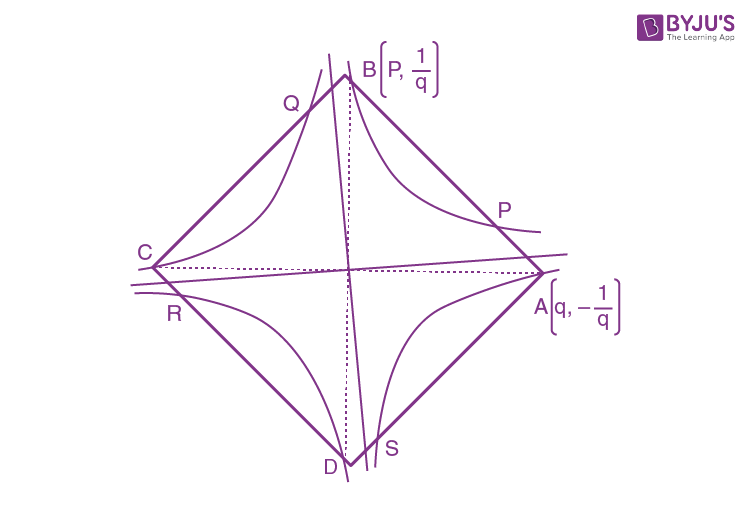OA ⟂ OB

(1 / p2) (- 1 / q2) = – 1

p2q2 = 1

p [(p + q) / 2, [(1 / p) – (1 / q)] / 2] lies on x2 y2 = 1

(p + q)2 [(1 / p) – (1 / q)]2 = 16

⇒ (p + q)2 (p – q)2 = 16

⇒ (p2 – q2)2 = 16

p2 – (1 / p2) = ± 4

⇒ p4 ± 4p2 – 1 = 0

p2 = (± 4 ± √20) / 2 = ± 2 ± √5

p2 = 2 + √5 or – 2 + √5

OB2 = p2 + (1 / p2) = 2 + √5 + 1 / (2 + √5) or – 2 + √5 + 1 / (- 2 + √5) = 2√5

Area = 4 (1 / 2) OA OB = 2 (OB)2 = 4 √5

Question 26: The missing value in the following figure is ______.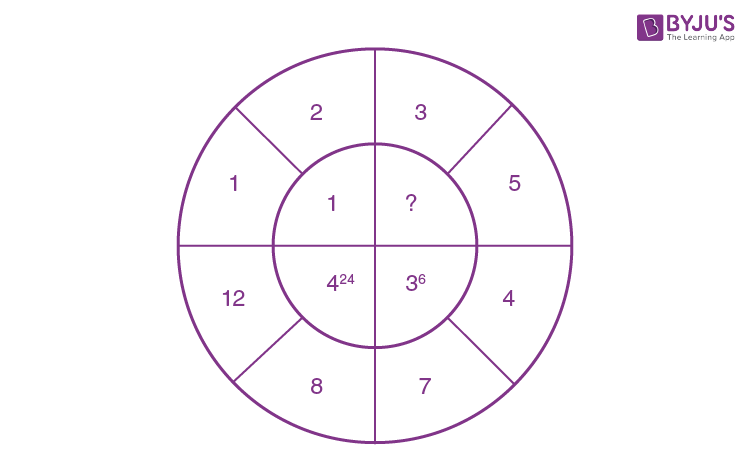424 has base 4 (= 12 – 8)

36 has base 3 (= 7 – 4)

(?) will have base 2 (= 5 – 3)

Power 24 = 6 × 4 = (no. of divisor of 12) × (no. of divisor of 8)

Power 6 = 2 × 3 = (no. of divisor of 7) × (no. of divisor of 4)

(?) will have power = (no. of divisor of 3) × (no. of divisor of 5) = 2 × 2 = 4

Question 27: The number of solutions of the equation |cot x| = cot x + (1 / sin x) in the interval [0, 2π] is ______.

Case I : x ∈ [0, π / 2] ⋃ [π, 3π / 2]

cot x = cot x + (1 / sin x) ⇒ not possible

Case II : x ∈ [π / 2, π] ⋃ [3π / 2, 2π]

– cot x = cot x + (1 / sin x)

– 2 cosx / sinx = 1 / sinx

cosx = – 1 / 2

x = 2π /3

= 1

Question 28: Let z1, z2 be the roots of the equations z2 + az + 12 = 0 and z1, z2 form an equilateral triangle with origin. Then, the value of |a| is ______.

In equilateral Δ,

z12 + z22 + z32 = z1z2 + z2z3 + z1z3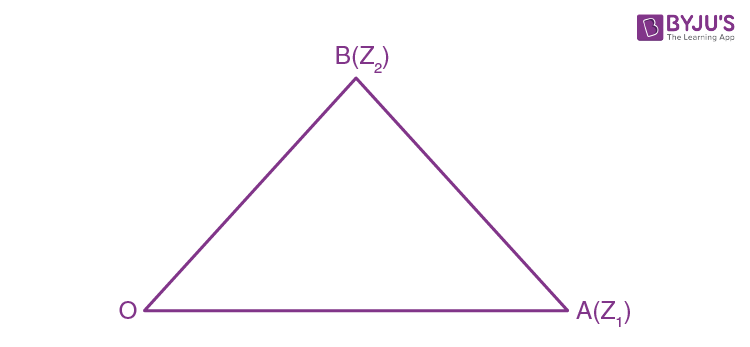z12 + z22 = z1z2 [because z3 = 0]

(z1 + z2)2 = 3z1z2

a2 = 36

|a| = 6

Question 29: Let the plane ax + by + cz + d = 0 bisect the line joining the points (4, –3, 1) and (2, 3, –5) at the right angles. If a, b, c, d are integers, then the minimum value of (a2 + b2 + c2 +d2) is ______.

The normal of plane = PQ = – 2i + 6j – 6k

a = –2, b = 6, c = –6 &

The equation of plane is – 2x + 6y – 6z + d = 0.

M (3, 0, – 2)

d = –6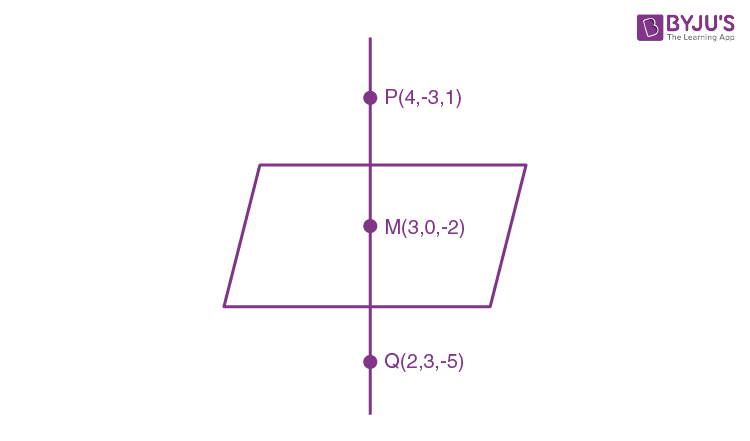Now equation of plane is – 2x + 6y – 6z – 6 = 0

x – 3y + 3z + 3 = 0

⇒ (a2 + b2 + c2 + d2)min = 12 + 9 + 9 + 9 = 28

Question 30: If f (x) = ∫ [5x8 + 7x6] / [x2 + 1 + 2x7]2 dx, (x ≥ 0), f (0) = 0 and f (1) = 1 / k, then the value of K is ______.

∫ [5x8 + 7x6] / [x2 + 1 + 2x7]2 dx

= ∫ [5x8 + 7x6] / [x14 {(2 + (1 / x5) + (1 / x7)}2] dx

∫ [(5 / x6) + (7 / x8)] / [(2 + (1 / x5) + (1 / x7)2] dx

Put (2 + (1 / x5) + (1 / x7) = t

= – [(5 / x6) + (7 / x8)] dx = dt

∫ (- dt / t2) = (1 / t) + c

f (x) = 1 / [2 + (1 / x5) + (1 / x7)] + c = [x7] / [(2x7 + 1 + x2)] + c

f(0) = 0 ⇒ C = 0

f (x) = 1 / 4 = 1 / k

⇒ k = 4